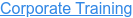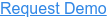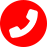# Anaplan Interview Questions and Answers

by Nithyanandham, on Dec 22, 2022 4:24:55 PM

## Ans

• Model Building
• Dynamic Scenario Building
• Model Versioning
• Dashboards & Reports
• Model Replication
• App Hub
• Calculation Engine
• API Integration
• Audit Trail
• Role-Based Access Settings
• Data Synchronization
• Master Data Repository
• Import/Export Capabilities
• Breakback
• Collaborative Approval Workflow
• In-Memory Processing

## Ans

The main benefits of Anaplan are its incredible features that help businesses make informed decisions, capabilities that support collaborative planning, a flexible and powerful in-memory engine, and intuitive and user-friendly interface.

## Ans

Anaplan is a comprehensive and robust organizational planning platform that aids big companies and organizations in making important, intelligent business plans and decisions by allowing them to accumulate and access significant business data easily. This software analyzes business performance data, which the decisionmakers or managers can employ when moving forward. The software generally covers enterprise-wide processes and single usages. With Anaplan, businesses and organizations can make sure that everyone involved in the decision-making is on the same page. It allows decision-makers to collaborate with ease, resulting in a faster process of finding resolutions to issues at hand.

## Ans

Modules are the components of each Anaplan model. These are comprised of line-items, timescales, list dimensions, and pages.

## Ans

Anaplan's modular approach lets decision-makers in Sales, Finance, Supply Chain, HR, Marketing, and other business units model their unique organizational structure. But you can also connect these models to compare, connect and calculate the relationships between them.## Ans

Anaplan chose to engage Google Cloud Professional Services for building two customer prototypes that used ML to enhance predictive planning methods.

## Ans

Numbered lists contain list items with unique identifiers. Create any lists you might need in General Lists in the model settings bar. When you convert a list to a numbered list, existing list items change to numbers. Learn how to preserve list item names before you convert a list.

## Ans

Anaplan's connected planning platform enables organizations to accelerate decision-making by connecting data, people, and plans across the business.

## Ans

To import into a module with a numbered list:

1. Create a line item in your source module to contain codes for each list item.
2. Type Code (Item(Listname)) in the line item's formula editor. Learn more in CODE.
Note: Listname is the name of your numbered list.
3. Select Pivot and pivot the line item to Columns, then select OK.
4. Select View > Save to save the module as a view.
5. In your target module, select Data > Import > Connect to Anaplan Model > Saved View, then select your view.
6. Map the Code property to the line item that contains the codes for each list item, then select Code as the unique identification method.
7. Select Run Import.

## Ans

Title

Description

ALL

The ALL aggregation function returns a TRUE result for all values that match specific Boolean criteria in a source module.

ANY

The ANY aggregation function returns a TRUE result for any value that matches specific Boolean criteria in a source module.

AVERAGE

The AVERAGE aggregation function takes a set of values from a source module and returns the mean average in a result module.

FIRSTNONBLANK

The aggregation function FIRSTNONBLANK returns the first value of a line item found for a given list item or time period.

LASTNONBLANK

The aggregation function LASTNONBLANK returns the last value of a line item found for a given list item or time period.

MAX

The MAX aggregation function returns the maximum value from a line item in a source module.

MIN

The MIN aggregation function returns the minimum value from a line item in a source module.

SUM

The SUM aggregation function sums values in a result module based on mapping from a source module.

TEXTLIST

The TEXTLIST aggregation function returns a collection of text values as a comma-separated value. The values returned are based on mapping from a source module.

## Ans

3. Click Access Control > Assignments.
4. Select the user.
5. Select the Page Builder checkbox.
6. Click Save.

## Ans

Title

Description

AGENTS

The AGENTS function calculates the number of servers (or agents) needed to fulfil requests within a target time.

AGENTSB

The AGENTSB function calculates the number of servers required to answer a specified percentage of calls (or SLA) within a busy period.

The ANSWERTIME function calculates the minimum hold time required to answer a certain percentage of calls, or service level agreement (SLA).

ARRIVALRATE

The ARRIVALRATE function calculates the maximum interval between requests possible while processing a specified percentage of these requests.

AVGDURATION

The AVGDURATION function calculates the required average duration of calls in order to answer a certain percentage of calls, or service level agreement (SLA).

AVGWAIT

The AVGWAIT function calculates the average waiting time for a request or call to be processed.

ERLANGB

The ERLANGB function determines the probability of a request being blocked given a specified number of servers, arrival rate of requests, and the average service duration.

ERLANGC

The ERLANGC function determines the probability of a request being placed in a queue given a specified number of servers, arrival rate of requests, and the average duration to process requests.

SLA

The SLA function calculates what percentage of calls must be answered within a target answer time, or service level agreement (SLA).

## Ans

Title

Description

COUPDAYBS

Use the COUPDAYBS (coupon days before settlement) function to calculate the number of days from the beginning of the coupon period until its settlement date. The number returned includes both the first day of the period and the settlement date.

COUPDAYS

Use the COUPDAYS function to return the number of coupon days in the coupon period that contains the settlement date.

COUPDAYSNC

Use the COUPDAYSNC function to calculate the number of coupon days from the settlement date until the next coupon date. The number returned excludes the settlement date and includes the last day of the next coupon period.

COUPNCD

The COUPNCD function calculates the next coupon date after a settlement date.

COUPNUM

The COUPNUM function returns the number of coupons payable between a settlement and maturity date.

COUPPCD

The COUPPCD function calculates the previous coupon date before a settlement date.

CUMIPMT

The CUMIPMT function calculates the cumulative interest paid on a loan over a period given equal payments made to the balance.

CUMPRINC

The CUMPRINC function calculates the amount of principal paid on a loan over a period, given consistent, equal payments.

DURATION

You can use the DURATION function to calculate the Macauley duration for an assumed parity value of 100 monetary units.

The Macauley duration is the weighted average maturity of cash flows. That is, the weighted average distance to payment. It's used to measure a bond price's response to changes in yield. A higher Macauley duration value indicates a riskier investment.

FV

The FV function calculates the future value of an investment. The future value is the lump sum or closing balance received at the end of an investment.

IPMT

The IPMT function calculates the amount of interest to be paid on a loan in a given payment period. The function assumes a consistent interest rate and payment timings in each period.

IRR

The IRR function calculates the internal rate of return for a series of positive and negative transactions. It can be used either with all transactions over a timescale, or with specified transactions on certain dates.

MDURATION

You can use the MDURATION function to calculate the modified Macauley duration for an assumed parity value of 100 monetary units.

The modified Macauley duration expresses the measurable change in the value of a bond in response to a change in interest rates. The result represents the effect that a 1% change in interest rates will have on the price of a bond.

NPER

The NPER function calculates the required number of periods to achieve a certain value for a loan or investment. This is based on a given interest rate, consistent payments, and opening and closing balance.

NPV

The NPV function calculates the net present value for a series of positive and negative transactions with a constant interest rate.

PMT

The PMT function calculates the payments due for a loan or annuity over a specified number of periods, given a consistent interest rate and payment amount.

PPMT

The PPMT function calculates how much of a payment is allocated to its principal part rather than interest. The function assumes a consistent interest rate and payment timings in each period.

PRICE

The PRICE function calculates the price per 100 monetary units invested for a bond that pays periodic interest.

PV

The PV function calculates the present value of an investment or the principal value of a loan.

RATE

The RATE function calculates the interest rate for a loan or investment based on length, payments, and present and future value.

YEARFRAC

Use the YEARFRAC function to calculate the fraction of a year between two dates (inclusive of the start date, exclusive of the end date).

The function uses a basis (day-count convention) to count the number of days between these dates, and then divide that number by the basis.

YIELD

Use this function to calculate the yield to maturity (YTM) of a bond.

## Ans

Title

Description

COMPARE

The COMPARE function compares text values. If they're the same, it returns 0. If the first text value is greater, it returns 1, and if the first text value is less, it returns -1.

IF THEN ELSE

Tests a Boolean argument and returns one of two results based on whether it is true or false.

ISACTUALVERSION

The ISACTUALVERSION function returns a TRUE result for the version that is set as Actual in a model. It returns FALSE for all other versions.

ISANCESTOR

The ISANCESTOR function takes two list or time period values. It returns a Boolean value of TRUE if the first is the ancestor of the second. An item is the ancestor of another if it is at a higher level of a list hierarchy.

ISBLANK

The ISBLANK function returns true for values that are blank.

ISCURRENTVERSION

The ISCURRENTVERSION function returns a TRUE result for the version that is set as Current in a model. It returns FALSE for all other versions.

ISFIRSTOCCURRENCE

The ISFIRSTOCCURRENCE function returns a Boolean value of TRUE for the first occurrence of a value in a list dimension.

ISNOTBLANK

The ISNOTBLANK function returns a Boolean result for values that are not blank.

LOOKUP

Use the LOOKUP function to look up values in a source module or list and display the values in a target module.

SELECT

Use the SELECT function to return values from a given list item or time period.

## Ans

Title

Description

CODE

The CODE function returns a list item's code.

COLLECT

Use the COLLECT function in a module that includes a line item subset to pull the source line item values into the module.

CURRENTVERSION

The CURRENTVERSION function returns the value from another line item for the version that is set as Current in a model.

FINDITEM

The FINDITEM function searches for a text value within the names and codes of the items or time periods in a given list or Time respectively. If the FINDITEM function finds a match, it returns the corresponding list item or time period.

ITEM

When used with a list, the ITEM function returns the list item that applies to each cell. When used with Time, it returns the time period that applies to each cell.

NEXTVERSION

The NEXTVERSION function evaluates the given expression using the next version.

PARENT

The PARENT function returns the parent item of list items and time periods.

PREVIOUSVERSION

The PREVIOUSVERSION function evaluates the given expression using the previous version.

RANK

The RANK function evaluates a set of values and assigns sequential rankings starting at 1.

RANKCUMULATE

The RANKCUMULATE function ranks values and then cumulates values in order of the ranking. It can perform ranking separately across different groups.

VALUE

The VALUE function converts text values that represent numbers to numbers.

## Ans

Title

Description

ABS

The ABS function returns the absolute value of a number. The absolute value of zero or a positive number remains the same. The absolute value of a negative number is the same number without the negative sign (the positive version).

DIVIDE

Use DIVIDE to divide one number by another.

EXP

The EXP function raises the mathematical constant e, or Euler's number, to the power you specify.

FIRSTNONZERO

The FIRSTNONZERO function searches through two or more numeric arguments and returns the first value that is not zero.

LN

Use the natural logarithm (LN) to work out the length of time it takes to achieve a unit of growth.

LN returns the natural logarithm of a number, based on the constant e. This function is the inverse of the EXP function, which raises e to the nth power.

LOG

The LOG function returns the logarithm of a number to the base you specify.

MAX

The MAX function returns the maximum from a set of values. For a number, it returns the maximum value. For a date, it returns the latest date.

MIN

The MIN function returns the minimum from a set of values. For a number, it returns the minimum value. For a date, it returns the earliest date.

MOD

The MOD function returns the remainder when one number is divided by another, or modulo.

MROUND

The MROUND function rounds a value to the nearest multiple of a number.

POWER

The POWER function raises a number to the power you specify.

ROUND

The ROUND function rounds a value to a specified number of decimal places, an integer, or a power of 10.

SIGN

The SIGN function returns the sign of a number (whether it's positive, negative, or zero). The SIGN function returns 1 for positive numbers, 0 for zero, and -1 for negative numbers.

SQRT

The SQRT function calculates the square root of a number.

## Ans

Title

Description

FIND

The FIND function searches for the first occurrence of a text value within another one. If the text contains the specified characters, the function returns a number. This number indicates the position of the first occurrence of the text value searched for.

LEFT

Extracts a string of characters from text, starting from the left.

LENGTH

The LENGTH (or LEN) function returns the number of characters in a text string.

LOWER

The LOWER function converts text values to lowercase.

MAILTO

Use the MAILTO function to generate clickable links that send an email. You can specify recipients, subjects, and body text.

MID

Extracts a number of characters from a text string, starting from a character you select.

NAME

Use the NAME function to convert data from a list item to text.

RIGHT

Extracts a string of characters from text, starting from the right.

SUBSTITUTE

The SUBSTITUTE function finds all occurrences of a text value within another one, and replaces them with a given value.

TEXT

The TEXT function converts numeric values to text.

TEXTLIST

The TEXTLIST function concatenates a series of text values into a single text value.

TRIM

The TRIM function removes all leading and trailing spaces, and extra spaces between words in a text string.

UPPER

The UPPER function converts text values to uppercase.